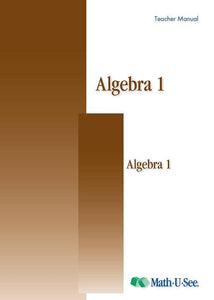or 6 weekly interest-free payments from \$14.16 withwhat's this?Demme Learning

# Math.U.See Algebra 1

Regular price \$20.00 \$48.83 Unit price per
Tax included. Shipping calculated at checkout.

## Math.U.See Algebra 1

Graphing, simultaneous equations, exponents, polynomials, unit multipliers, and more.

Major Concepts and Skills Include:

• Graphing linear equations and inequalities
• Describing linear equations in slope-intercept and standard form
• Solving systems of equations using graphing, substitution, and elimination
• Expanding understanding of exponents
• Solving basic quadratic equations with factoring
• Using unit multipliers to convert between units of measure

• Applying properties of arithmetic functions to algebra
• Adding, multiplying, and dividing polynomials
• Applying significant digits to computations with scientific notation
• Converting between base 10 and other bases
• Graphing conic sections
• Applying significant digits to computations with scientific notation